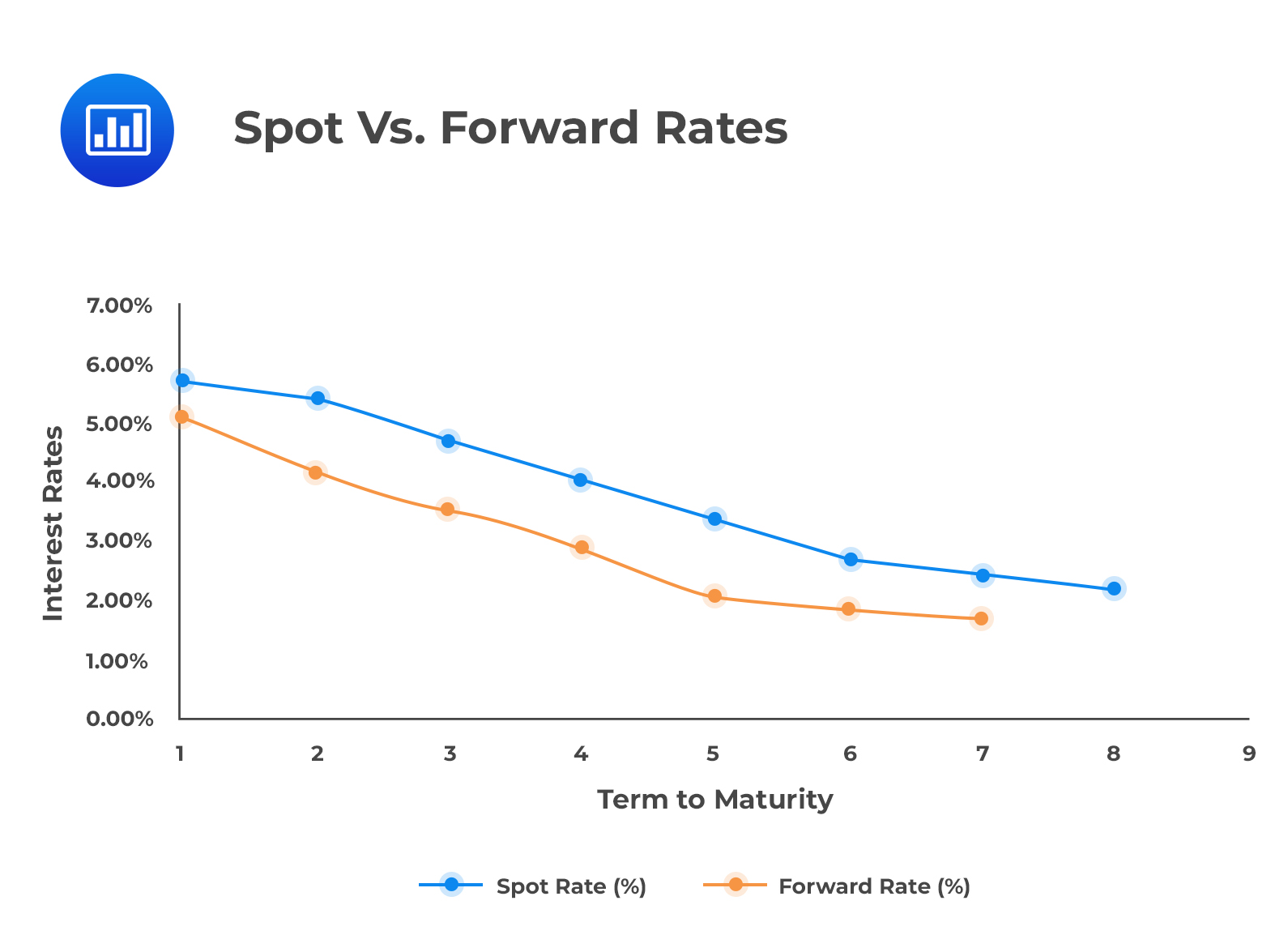# Assumptions Relating to the Evolution of Spot Rates

Recall from the first learning objective of this reading that the forward rate lies above the spot rate for an upward sloping spot curve. On the other hand, the forward rate in a downward sloping spot curve lies below the spot rate.

This relationship in a downward sloping spot curve is shown in the figure below:

###The Spot Rate for Long-Maturity Securities

The spot rate for a bond with a maturity greater than 1 is equal to the geometric mean of the one-period spot rate and a series of one-year forward rates.

From the forward rate model:

$$\left(1+S_T\right)^T=(1+S_1)\left[1+f\left(1,T-1\right)\right]^{T-1}$$

Which can be expanded to:

If $$t=1$$,

\begin{align*} & \left(1+S_T\right)^T \\ & =(1+S_1)(1+f(1,1)(1+f(2,1)(1+f\left(3,1\right)\ldots (1+f\left(T-1,1\right)\ldots({1}) \\ & S_T \\ & =[(1+S_1)(1+f(1,1)(1+f(2,1)(1+f\left(3,1\right)\ldots \\ & \left(1+f\left(T-1,1\right)\right]^\frac{1}{T}-1\ldots({2}) \end{align*}

Contrary to the unbiased expectations theory, active bond portfolio management suggests that forward rates are biased predictors of future spot rates. In other words, the forward curve does not accurately forecast future spot rates.

Thus, an active portfolio manager tries to outperform the bond market by forecasting how the future spot rates will differ from those reflected by the current forward curve.

#### Example: Spot and Forward Prices and Rates

The following information relates to three zero-coupon bonds.

$$\begin{array}{c|c} \textbf{Maturity} & \textbf{Spot Rates} \\ \hline 1 & 10\% \\ \hline 2 & 11\% \\ \hline 3 & 12\% \end{array}$$

1. Calculate the implied one-year forward rate for a zero-coupon bond issued one year from today (i.e., f (1,1)).
2. Calculate the implied one-year forward rate for a zero-coupon bond issued two years from today (i.e., f (2,1)).
3. Calculate the two-year spot rate and the three-year spot rate assuming that the spot rates evolve as implied by the current forward curve.

Solution

1. $$f (1,1)$$

\begin{align*} (1+f\left(1,1\right)) &=\frac{\left(1+S_2\right)^2}{\left(1+S_1\right)} \\ f\left(1,1\right) & =\frac{{1.11}^2}{1.10}-1=1.1201-1=12.01\% \end{align*}

2. $$f (2,1)$$

\begin{align*} (1+f\left(2,1)\right) &=\frac{\left(1+S_3\right)^3}{\left(1+S_2\right)^2} \\ f\left(1,2\right) & =\frac{{1.12}^3}{{1.11}^2}-1=14.03\% \end{align*}

3. Geometric averages

\begin{align*} \left(1+S_2\right)^2 & =(1+S_1)(1+f(1,1)) \\ S_2 & =\left[\left(1+0.10\right)\left(1+0.1201\right)\right]^\frac{1}{2}-1\approx11\% \\ \left(1+S_3\right)^3 & =[\left(1+S_1\right)(1+f(1,1)(1+f\left(2,1\right)] \\ S_3 &=\left[\left(1.10\right)\left(1.1201\right)\left(1.1403\right)\right]^{\left(\frac{1}{3}\right)}-1 \\ & =\approx12\% \end{align*}

## Question

A bond whose expected spot rate is lower than the current forward rate is most likely:

1. Undervalued.
2. Overvalued.
3. Fairly valued.

### Solution

The bond’s expected price determined using a lower expected discount rate (spot rate) is higher than the price obtained using the current forward rate discount factor. This implies that the bond will be undervalued.

Reading 28: The Term Structure and Interest Rate Dynamics

LOS 28(c) Describe the assumptions concerning the evolution of spot rates in relation to forward rates implicit in active bond portfolio management.

Shop CFA® Exam Prep

Offered by AnalystPrepLevel I
Level II
Level III
All Three Levels
Featured Shop FRM® Exam PrepFRM Part I
FRM Part II
FRM Part I & Part II
Learn with Us

Subscribe to our newsletter and keep up with the latest and greatest tips for success
Shop Actuarial Exams PrepExam P (Probability)
Exam FM (Financial Mathematics)
Exams P & FMGMAT® Complete CourseDaniel Glyn
2021-03-24
I have finished my FRM1 thanks to AnalystPrep. And now using AnalystPrep for my FRM2 preparation. Professor Forjan is brilliant. He gives such good explanations and analogies. And more than anything makes learning fun. A big thank you to Analystprep and Professor Forjan. 5 stars all the way!michael walshe
2021-03-18
Professor James' videos are excellent for understanding the underlying theories behind financial engineering / financial analysis. The AnalystPrep videos were better than any of the others that I searched through on YouTube for providing a clear explanation of some concepts, such as Portfolio theory, CAPM, and Arbitrage Pricing theory. Watching these cleared up many of the unclarities I had in my head. Highly recommended.Nyka Smith
2021-02-18
Every concept is very well explained by Nilay Arun. kudos to you man!2021-02-13